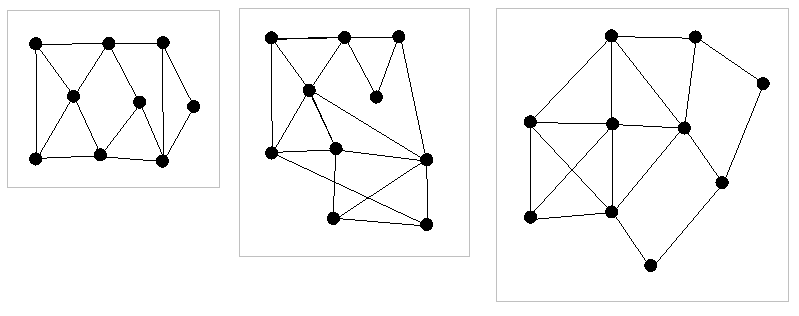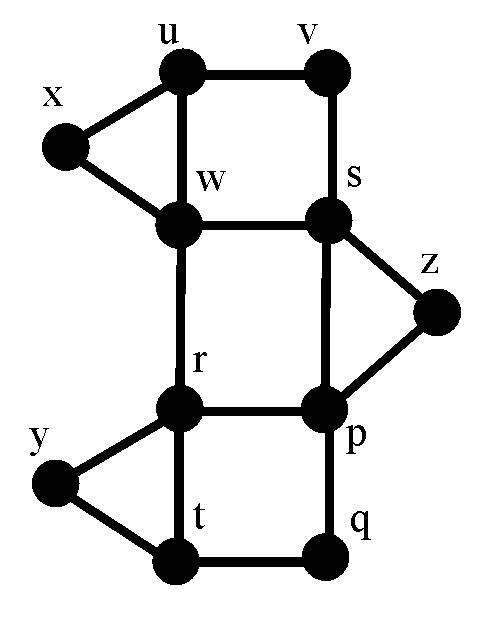# Midterm

## 2.1

1: Explain the Pigeonhole principle

 79%
If you divide n items into m categories, and n > m, then at least one category must contain two or more items.

2a: Assume 1200 people are in the same room. How many people can you guaranteed find that celebrate their birthday on the same day?
2b: Assume 1500 people are in the same room. How many people can you guaranteed find that celebrate their birthday on the same day?

 73%
a) Since 1200/365 is greater than 3, there must be a day where at least 4 have birthday. b) Since 1500/365 is greater than 4, there must be a day where at least 5 have birthday. Note that these results have nothing to do with probabilty, we are certain that such a day exists. It's a guarantee.

## 2.2

3a: List the first ten Fibonacci numbers

 100%
1, 1, 2, 3, 5, 8, 13, 21, 34, 55, 89, ...

3b: What has the Golden ratio to do with Fibonacci numbers?

 75%
The ratios of consecutive Fibonacci numbers are approaching the golden ratio.

4a: Compute the value of the following compound fraction:4b: Compute the value of the following compound fraction:63%
A: 5/3
B: 2/3

5a: Write the number 60 as a sum of distinct nonconsecutive Fibonacci numbers.
5b: Write the number 70 as a sum of distinct nonconsecutive Fibonacci numbers.

 90%
A: 60 = 55 + 5.
B: 70 = 55 + 13 + 2.

## 2.3

6a: Write the number 130 as a product of prime numbers.
6b: Write the number 126 as a product of prime numbers.

 67%
A: 130 = 2 · 5 · 13.
B: 126 = 2 · 3 · 3 · 7.

7a: Who gave the first proof that there are infinitely many prime numbers?.

 0%
Euclid.

7b: How many prime numbers are there?.

 83%
Infinitely many.

8: Is the number 123 + 12311 prime? Why or why not?

 18%
123 + 12311 has a factor of 123, 123 + 12311 = 123(1+ 12310).

## 2.4

9a: Reduce 3·5·7·11·13+1 modulo 11. (Meaning, find that number among 0,1,2,...10 that is equivalent to 3·5·7·11·13+1 (mod 11).)
9b: Reduce 3·5·7·11·13+1 modulo 7. (Meaning, find that number among 0,1,2,...6 that is equivalent to 3·5·7·11·13+1 (mod 7).)

 38%
A: Remember that if you make a computation modulo 11, you can always replace a number by another one that is equilvalent modulo 11 and get a result equivalent to the previous one modulo 11. Therefore, since 11 ≡ 0 (mod 11), you con replace the 11 by 0 and obtain 3·5·7·0·13+1 = 1.
B: In the same way 3·5·7·11·13+1 ≡ 3·5·0·11·13+1 = 1 (mod 7).

10a: It is Monday today. What day will there be in 789 days?
10b: It is Monday today. What day will there be in 779 days?

 50%
For (a) it's Saturday, since 789 = 784+5 = 7 · 112 + 5 ≡ 5 (mod 7).
For (b) it`s Wednesday, since 779 = 777+2 = 7 · 111 + 2 ≡ 2 (mod 7).
Here I was also disappointed. That is the reason for introducing the concept of modulo n. Questions like "It is 3 o clock in the afternoon---what times is it in 800 hours?" Everybody in this class should know how to do this.

11: When are two numbers "equivalent modulo 11"?

 30%
If their difference is a multiple of 11, or if both numbers leave the same remainder when dividing by 11.
This is the main concept of this section. Everybody should understand it, and should also be able to explain it.

## 4.2

12: What does the Art Gallery Theorem say?

 40%
In every art gallery with n vertices we can place at most n/3 guards at vertices such that they "see" the whole gallery. This is really the core of 4.2---without an understanding of what this statement says, all this triangulation and coloring procedures are in vain.

 13a: Find a 3-coloring of the vertices (such that every triangle has all three colors) of the following triangulation of an art gallery.13b: Find a 3-coloring of the vertices (such that every triangle has all three colors) of the following triangulation of an art gallery.88%
A: ....
B: ....

## 4.3

14: Explain a simple procedure how to produce another golden rectangle of different size from a given golden rectangle.

 75%
Remove a largest inscribed square. Or double all sides. Or fold in half twice, once horizontally and onc vertically.

## 5.4

15a: How many edges does a convex polyhedron with 20 vertices and 11 faces have?
15b: How many edges does a convex polyhedron with 15 vertices and 18 faces have?

 69%
A: Since V-E+F=2, and V=20 and F=11, we get E=29.
B: Since V-E+F=2, and V=15 and F=18, we get E=31.

16a: You have a polyhedron with 14 faces, all of them triangles. How many edges does the polyhedron have?
16b: You have a polyhedron with 16 faces, all of them triangles. How many edges does the polyhedron have?

 46%
Remember that the sum of all degrees of all faces equals twice the edge numbers. Triangles have degree 3. Thus
A: 3 · 14 = 2 · E, hence E=21.
B: 3 · 16 = 2 · E, hence E=24.

17a: Which one of the following three graphs is a plane graph?17b: Which one of the following three graphs is a plane graph?79%
A:: The third one.
B: The first one.

18: For the plane graph in the previous question, draw its dual.

 54%

19: Explain what Euler's polyhedron formula states about polyhedra. Who proved this result?

 45%
V-E+F = 2 for every convex polyhedron, where V, E, and F denote number of vertices, edges, and faces. This is the version Cauchy proved. There are other versions around, for other classes of polyhedra.

## 5.320: Does the graph to the right have an open or closed Eulerian tour? If it doesn't, why not, if it has, write it down (as a sequence of the vertices).

 35%
It has an open Eulrian tour, since two vertices have odd degree and all others even degree. It must start at one of the odd degree vertices and end at the other odd degree vertex. One possibility (among many) is u, x, w, y, v, s, w, r, p, z, s, p, q, t, r, y, t, using the edges ux, xw, wy, yv, vs, sw, wr, rp, pz, zs, sp, pq, qt, tr, ry, yt.